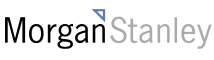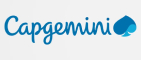New update is available. Click here to update.
Last Updated: 5 Nov, 2020

# Design a hashset

Moderate+2 more companies

## Problem statement#### Implement the following public functions :

``````1) Constructor: It initializes the data members as required.

2) add(value): It inserts an element into the HashSet. The function takes one argument which is the value that needs to be added and returns nothing

3) contains(value): It checks whether the element exists in the HashSet or not. The function takes one argument which is the value that needs to be searched for in the HashSet. The function returns true if the element exists, otherwise returns false.

4) remove(value): It removes an element from the HashSet. The function takes one argument which is the value that needs to be removed from the HashSet and returns the element which is being removed. If the element does not exist in the HashSet or if HashSet is empty, return -1.
``````
##### Operations Performed on the HashSet:
``````Query-1 (Denoted by an integer 1)- Inserts an element in the HashSet

Query-2 (Denoted by an integer 2)- Returns a boolean value denoting whether the element is present in the HashSet or not.

Query-3 (Denoted by an integer 3)- Removes the element from the HashSet.
``````
##### Input format:
``````The first line of input contains an integer ‘Q’ denoting the number of queries.
The next ‘Q’ lines represent the queries that need to be performed.

Each query case contains two integers, two integers separated by a single space, representing the type of the operation, and a value on which operation needs to be performed.
``````
##### Output format
``````For Query 1, you do not need to return anything.

For Query 2, return true or false depending upon whether the element is present or not.

For Query 3, return the element which is being removed from the HashSet.
``````
##### Note:
``````You do not need to print anything; it has already been taken care of. Just implement the given function.
``````
##### Constraints:
``````1 <= Q <= 10^3
1 <= query type <= 3
0 <= VALUE <= 10^6

Where ‘Q’ is the total number of queries, ‘value’ is the element that will be added, removed, or whose existence in the HashSet is to be checked.

Time limit: 1 second
``````## Approaches

### 01 ApproachThe idea is to store the elements in the hash table to reduce the space. To avoid a collision, chaining is used.

1. Make a hash table of size 1000.
2. For the addition of a value to a hash table, find its index by passing it in a hash function. In the hash function, the modulus of the value and a constant is taken. The result is the index where the element will be stored in the hash table.
3. To avoid a collision, use chaining. In chaining, each cell of the hash table points to a linked list of records that have the same hash function value. So, to add the element, just push the element in the list at the found index.
4. To remove an element, first find its index in the hash table, then search the element in the list of records present at that index. If the element is found, erase the element and return the removed element. If the element is not present already, return -1.
5. To search an element, first find its index in the hash table. Now, search for the element in the list of records present at the index. If the element is found, return true. Else return false.